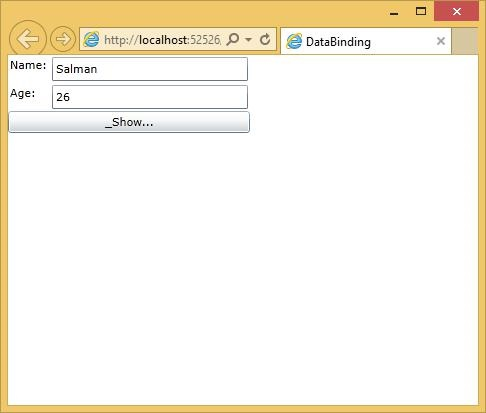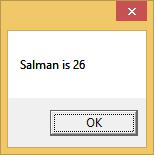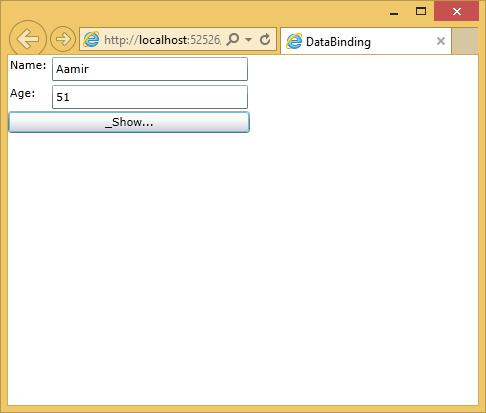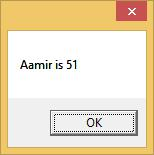# Silverlight - Data Binding

Data binding is a mechanism in Silverlight application, which provides a simple and easy way for Windows Runtime apps using partial classes to display and interact with data. The management of data is separated entirely, from the way data is displayed in this mechanism. Data binding allows the flow of data between UI elements and the data object on user interface. When a binding is established and the data or your business model changes, then it will reflect the updates automatically to the UI elements and vice versa. It is also possible to bind, not to a standard data source, but rather to another element on the page.

Data binding are of the following two types −

• One-way data binding
• Two-way data binding

## One-way Data Binding

In one-way data binding, the data is bound from its source (that is the object that holds the data) to its target (that is the object that displays the data).

Let us have a look at a simple example of one-way data binding.

Given below is the XAML code in which two labels, two text boxes and one button are created with some properties.

```<UserControl x:Class = "DataBinding.MainPage"
xmlns = "http://schemas.microsoft.com/winfx/2006/xaml/presentation"
xmlns:x = "http://schemas.microsoft.com/winfx/2006/xaml"
xmlns:d = "http://schemas.microsoft.com/expression/blend/2008"
xmlns:mc = "http://schemas.openxmlformats.org/markup-compatibility/2006"
mc:Ignorable = "d"
d:DesignHeight = "300" d:DesignWidth = "400">

<Grid x:Name = "LayoutRoot" Background = "White">

<Grid.RowDefinitions>
<RowDefinition Height = "Auto" />
<RowDefinition Height = "Auto" />
<RowDefinition Height = "*" />
</Grid.RowDefinitions>

<Grid.ColumnDefinitions>
<ColumnDefinition Width = "Auto" />
<ColumnDefinition Width = "200" />
</Grid.ColumnDefinitions>

<TextBlock Name = "nameLabel" Margin = "2">Name:</TextBlock>
<TextBox Name = "nameText" Grid.Column = "1" Margin = "2"
Text = "{Binding Name, Mode=OneWay}"/>

<TextBlock Name = "ageLabel" Margin = "2" Grid.Row = "1">Age:</TextBlock>

<TextBox Name = "ageText" Grid.Column = "1" Grid.Row = "1" Margin="2"
Text = "{Binding Age, Mode = OneWay}"/>

<StackPanel Grid.Row = "2" Grid.ColumnSpan = "2">
<Button Content = "_Show..." Click = "Button_Click" />
</StackPanel>

</Grid>

</UserControl>
```

We observe the following things −

• The text properties of both the text boxes bind to “Name” and “Age”, which are class variables of Person class as shown below.

• In Person class, we have just two variables Name and Age, and its object is initialized in MainPage class.

• In XAML code, we are binding to a property Name and Age, but we have not selected which property belongs to the object.

• An easy way is to assign an object to DataContext whose properties we are binding in the C# code in MainPage constructor as shown below.

```using System.Windows;
using System.Windows.Controls;

namespace DataBinding {

public partial class MainPage : UserControl {
Person person = new Person { Name = "Salman", Age = 26 };

public MainPage() {
InitializeComponent();
this.DataContext = person;
}

private void Button_Click(object sender, RoutedEventArgs e) {
string message = person.Name + " is " + person.Age;
MessageBox.Show(message);
}
}

public class Person {
private string nameValue;

public string Name {
get { return nameValue; }
set { nameValue = value; }
}

private double ageValue;

public double Age {
get { return ageValue; }

set {
if (value != ageValue) {
ageValue = value;
}
}
}
}
}
```

Let us run this application and you can see in your webpage immediately that we have successfully bound to the Name and Age of that Person object.When you press the Show button, it will display the name and age in the message box.Let us change the Name and Age in the above dialog box.Now, if you click the Show button, it will display the same message again.This is because the data-binding mode is set to oneway in the XAML code. To show the updated message, you will need to understand the two-way data binding.

## Two-way data binding

In two-way binding, the user is able to modify the data through the user interface and have that data updated in the source. If the source changes while the user is looking at the view, you want the view to be updated.

Let us have a look at the same example but only change the binding mode from one-way to two-way binding in XAML code as shown below.

```<UserControl x:Class = "DataBinding.MainPage"
xmlns = "http://schemas.microsoft.com/winfx/2006/xaml/presentation"
xmlns:x = "http://schemas.microsoft.com/winfx/2006/xaml"
xmlns:d = "http://schemas.microsoft.com/expression/blend/2008"
xmlns:mc = "http://schemas.openxmlformats.org/markup-compatibility/2006"
mc:Ignorable = "d"
d:DesignHeight = "300" d:DesignWidth = "400">

<Grid x:Name = "LayoutRoot" Background = "White">

<Grid.RowDefinitions>
<RowDefinition Height = "Auto" />
<RowDefinition Height = "Auto" />
<RowDefinition Height = "*" />
</Grid.RowDefinitions>

<Grid.ColumnDefinitions>
<ColumnDefinition Width = "Auto" />
<ColumnDefinition Width = "200" />
</Grid.ColumnDefinitions>

<TextBlock Name = "nameLabel" Margin = "2">_Name:</TextBlock>

<TextBox Name = "nameText" Grid.Column = "1" Margin = "2"
Text = "{Binding Name, Mode=TwoWay}"/>

<TextBlock Name = "ageLabel" Margin = "2" Grid.Row = "1">_Age:</TextBlock>

<TextBox Name = "ageText" Grid.Column = "1" Grid.Row = "1" Margin = "2"
Text = "{Binding Age, Mode = TwoWay}"/>

<StackPanel Grid.Row = "2" Grid.ColumnSpan = "2">
<Button Content = "_Show..." Click = "Button_Click" />
</StackPanel>

</Grid>

</UserControl>
```

Let us run this application again and you can see the same output.Let us change the Name and Age in the above dialog box.Now, if you click the Show button it will display the updated message.